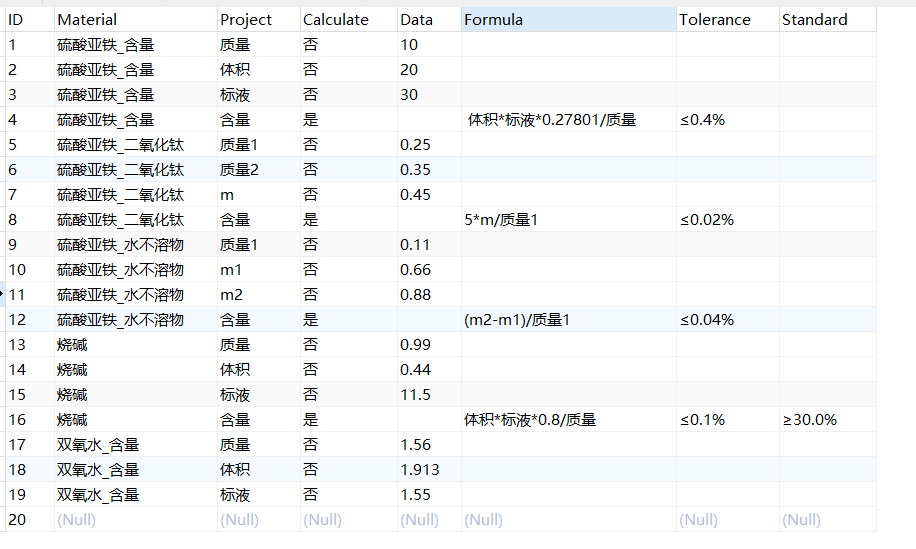Orcale合并重复的记录，并求出计算结果？？
`````` declare
--游标类型定义
cursor table_cur
is
select Material,Formula from Table_1
WHERE Calculate='是';
cursor table_str
IS
select projects,datass from  TABLE_1
where  calculate<>'是';
--定义一个游标变量table_row table_cur%ROWTYPE ，该类型为游标table_cur中的一行数据类型
table_row table_cur%rowtype;
table_cow table_str%rowtype;
Formula table_1.Formula%type;

begin
--游标for循环开始
for table_row in table_cur loop
for table_cow in table_str loop

Formula:=replace(table_row.Formula,table_cow.Projects,table_cow.Datass);

dbms_output.put_line(Formula);
end loop;
end loop;

----游标for循环结束
end;
``````根据公式 替换数值 求出 Datass的值,,,

1个回答

cursor table_cur
is
select Material,Formula from Table_1
WHERE Calculate='是';
cursor table_str
IS
select projects,datass from TABLE_1
where calculate<>'是';